Experience is a great teacher. And in my experience, the beginning has always been tough.

As a BI consultant & trainer, I have worked with various people: from the frontline employees to CEOs. Our interactions gave me great insights into the multiple challenges they face.

I have put together a few pages from my experience and learning; covering Power BI, Excel, Power Query, Power Pivot, Tableau, data analytics & data visualization.

The purpose of the blog is to get you started.

I hope you’ll find them helpful.

Vivek

SearchHow many of you use Nested IFs in Excel? Mastering Nested IFs takes time and effort, especially those who are not savvy with formulas. Somehow you managed to write Nested IFs, but when it comes to editing or making modifications, then it looks like a challenge—struggling with proper brackets and conditions. Complicated at times :)

Well, there is good news. Modern Excel (Office 365 & Excel 2016 -19) has tamed this beast in multiple ways. In this article, we are going to see a couple of them.

I am hoping that you are familiar with IF.

This function follows a typical IF (Condition is TRUE) THEN (Result/Action 1) ELSE (Result/Action 2) logic. In the case of multiple conditions, we must use the IF function multiple times. Let us take a simple example:

Following table stands for the divisions based on the marks scored in the exam:## Nested IFs using AND (The traditional approach)

In traditional Excel, the following would have been the possible solution:

`=IF(A2<35,"Fail",IF(AND(A2>=35,A2<45),"3rd",IF(AND(A2>=45,A2<60),"2nd","1st")))`Question: How many "IF" needed in the traditional nested ifs?

The thumb rule is: If the number of categories is n, then if need to supply (n-1) IF. In this example, there are four categories (Fail, 3rd, 2nd, and 1st). Hence, we need (4-1) = 3 IF. The last category comes under else output of the last IF statement.

This approach is a little complicated, especially those who are relatively new at Excel.

## Nested IF using IFS (The modern approach)

In Modern Excel, we have something called IFS and here how it has replaced the traditional IF:

`=IFS(A8<35,"Fail",A8<45,"3rd Division",A8<60,"2nd Division",A8>=60,"1st Division")`IFS function is 23% shorter than the traditional Nested IF (in terms of length of the formula) and relatively easy to write. No need to worry about combining IF, AND, and OR.

One thing which IFS required is that you cover all the ground of logic. In this example, we need to tell IFS function what value to return for Marks Scored >= 60 as well. It was not the case in the traditional IF statement.

## Nested IF using LOOKUP (The smart approach)

There is another smart way to handle such a scenario: using LOOKUP. Not VLOOKUP or HLOOKUP, but just LOOKUP.

All you need to do is create a simple table with the threshold defined for each level (as shown in the table below) and then apply the formula at the desired cell:

`=LOOKUP(A2,\$G\$3:\$H\$6)`Voila! That's it.

LOOKUP has two segments

LOOKUP_VALUE: The cell having the value. In this example, A8 is the cell having the mark

ARRAY: The range of the table. In this example, G3:H6. Do not include the header.

You need to take care of the following points:

· The threshold should be the start point of the level. So, in this example, the Fail starts from 0, 3rd division starts from 35, so on and so forth.

· Fix the table range by using \$ signs as there is a high probability that you would be copy-pasting this formula in multiple cells. In this example, I have fixed the array A2:B5 to \$A\$2:\$B\$5

## Nested IF using XLOOKUP (The smarter approach)

One of the latest entrants in formula and arguably very versatile. It has gained significant popularity among Excel users due to a wide range of options it offers.

XLOOKUP has the following syntax:

Lookup_value: What you are looking for

Lookup_array: Where you are looking for

Return_array: The output column you need if the match found

If_not_found (optional): What to return if no matches found

Match_mode (optional): By default, it selects "0-Exact Match", but it offers the following added options:So, our formula is:

`=XLOOKUP(A2,\$H\$3:\$H\$6,\$I\$3:\$I\$6,,-1)`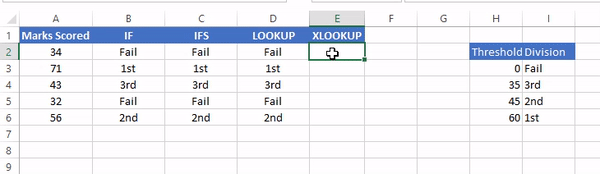Applying XLOOKUP for distributing data into categories

XLOOKUP advantage over LOOKUP: LOOKUP requires the reference table to sorted in ascending order for correct output, while XLOOKUP doesn't.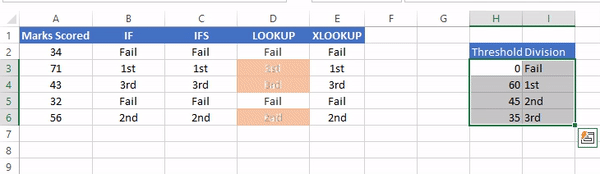So, which one do you prefer?

Related article: Adding Categories with Power Query

@imVivRan

## DAX Filter Context & Row Context: What are they?DAX is a simple language. You can start using DAX in a matter of hours. When it comes to an understanding of the advanced concepts like evaluation contexts, iterations, and context transitions, life with DAX becomes tricky. Especially for those whose most significant coding achievement is writing some Excel formulas. I was one of them.

After a while, I understood that one needs to be patient with it. It just takes some time and practice getting used to along with finding the right resource for reference. I found it in the form of a book: The Definitive Guide to DAX (by Marco Russo and Alberto Ferrari)

After going through the book, I realized that to unleash DAX's full power, a sound understanding of the evaluation context is essential.

The following are the excerpts from the book, along with my understanding.

## What is the evaluation context?

The evaluation context is the "environment" within which the DAX calculates the expression.

There are two evaluation contexts in DAX: The Filter context and the Row context.

The filter context filters data, and the row context iterates table.

DAX evaluates all formulas within a respective context. Even though the equation is the same, the result is different because DAX executes the same code against different subsets of data.

We use DAX for writing Measures and Calculated Columns. A DAX measure evaluates in the context of a visual element, or a DAX query. A Calculated Column processed at the row level of the table it belongs to.

## Understanding Filter Context:

Consider a simple measure:

``` Total Orders = SUM(Orders[Order Quantity])
```

This formula computes the sum of column Order Quantity. We can use this measure in a visual to get the result:The output above looks obvious, but precisely this is what we asked DAX to do.

We can further slice this value by different dimensions (let’s say, Product Category) to get interesting insights:Although it is intuitive, instead of showing the total sum of Order Quantity, it is now giving the sum of sales for each product category along with the total. We did not apply the filter in the DAX. This filtering happens outside of the formula.

Essentially, each cell in the visual is creating a filter context(environment) and filter the table by each Product Category and then applying the SUM function on each filter context.

The DAX engine might have some internal optimization to improve speed, but every cell has an independent and autonomous evaluation of underlying DAX expression.

Hence, the Total row in the table above is not a result of summing other cells in the visual.

It is the aggregation of all the rows of the Order table.

Depending on the DAX expression, the total row's result might display a different result, unrelated to the other rows in the same report.

When the Product Category is on the rows, the filter context filters one product category for each cell. We can increase the complexity of the visual by adding Ship Mode on the columns:Each cell shows a subset of data related to one product category and one ship mode. Now the filter context is filtering the Order table by each Product Category and Ship Mode, and then applying the DAX expression.

The rule is simple: The more dimensions we use to slice and dice, the more columns are being filtered by the filter context in each cell of the matrix visual.

Whether a dimension is on the rows or the columns of the visual, the slicer, part of any filter(visual/report/page), or any other kind of filter we can create with a report doesn't change the fundamental behavior of filter context. All these filters contribute to defining a single filter context that DAX uses to evaluate the formula.

Filter context filters (tables).

### Understanding Row Context

Row context iterates over tables and evaluates column values. The simplest way of understanding a row context is to create a calculated column. Consider the following DAX expression:

``` Sales Amount = Orders[Order Quantity] * Orders[Unit Price]
```

The expression above creates an additional column with a different value for each row:Though, this behavior is expected, especially by Excel users, where native Excel tables display similar behavior. It comes as a natural consequence that the final expression computes different values.

As it happened with the filter context, the reason is the presence of an evaluation context. This time, the context does not filter a table. Instead, it identifies the row for which the calculation happens.

The row context references a row in the result of a DAX table expression. It should not be confused with a row in the report. DAX does not have a way to reference a row or a column in the report directly.

The values displayed in a matrix in Power BI and a Pivot Table in Excel are the results of DAX measures computed in a filter context or the values stored in the table as native or calculated columns.

We realized that a calculated column is computed row by row, but how does DAX know which row it is currently iterating? It does because of the row context, which provides the evaluation context(environment) as a row.

With the creation of a calculated column with multiple records present in the table, DAX creates a row context that evaluates the expression iterating over the table by row, using the row context as the cursor.

When we create a calculated column, DAX creates a row context automatically. How can we create the row context manually? The answer is by using iterators.

``` Sales Amount (Measure) =
SUMX(
Orders,
Orders[Order Quantity] * Orders[Unit Price]
)

```

In this case, since it is a measure, there is not an automatic row context. SUMX, being an iterator, creates a row context that starts iterating over the Order table, row by row. During the iteration, it executes the second argument of SUMX insider the row context.

It is how the DAX knows which value to use for the two columns used in the expression during each step of the iteration.

Row context exists when:

• Creating a calculated column

• Computing an expression inside an iteration

There is no other way of creating a row context.

``` Sales Amount = Orders[Order Quantity] * Orders[Unit Price]
```

The expression above is valid when executed as a calculated column and invalid if used in a measure. The reason is that the calculated column has an automatic row context, whereas a DAX measure needs an iterator to create a row context.

Iterators in DAX follow below-mentioned steps:

• Evaluates the first parameter in the existing context(read filter contexts) to determine the rows to scan

• Creates a new row context for each row of the table evaluated in the previous step

• Iterates the table and evaluates the second parameter in the existing evaluation context, including the newly created row context.

• And last, aggregates the values computed during the previous step.

DAX Iterators: The X-factor

To summarize: A row context is not filtering the model in any way. It only tells the DAX which row to use from the table. If one wants to apply a filter to the model, then we use the filter context. In the case of row-level calculation, we use the row context.

Filter context filters, row context iterates.

@imVivRan

## How to FILTER in Excel formulas?In my early days of MS Excel, VLOOKUP was quite popular. It used to be a proud arsenal for one who is used Excel in their day-to-day work. But at times, that wasn’t enough. We wished if we could add additional filters to the VLOOKUP function. The technology was not ready then.

The past few years have been quite exciting. Microsoft has made Excel a versatile package: storing, processing & modeling data has become a more straightforward task. Inclusion of new functions, Power Query, Power Pivot, and new graphs like Box Plots, Histograms, Tree Maps, and more have made Excel quite formidable application. And finally, we have a function that allows us to include additional filters.

## Introducing FILTER

Quite a simple function, FILTER function has the following syntax:

FILTER (array, include,[if empty])

· Array: The range which we need in return

· Include: The column which we would like to filter

· [if empty] (Optional): Values to return if no matches found

The output of the FILTER function is an array that meets the criteria defined under the “Include” argument.

Let us consider the example below: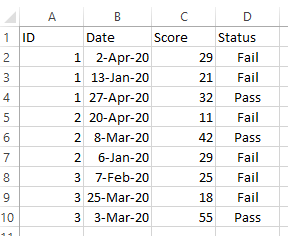We can use the following formula in cell F5

`=FILTER(A1:D10,D1:D10=F3,"")`And it returns a table with the matching Status (taking reference from cell F3)

Gif image

FILTER function can also return a single column:

`=FILTER(C1:C10,D1:D10=F3,"")`And it returns a table with the matching Status (taking reference from cell F3)FILTER function can also return a single column:

`=FILTER(C1:C10,D1:D10=F3,"")`## FILTER with AND criteria

We can combine multiple conditions using the special character “* “ in the Include argument:

`=FILTER(A1:D10,(D1:D10="Fail") * (C1:C10<25),"")`

It returns an array where the Status = Fail AND Score < 25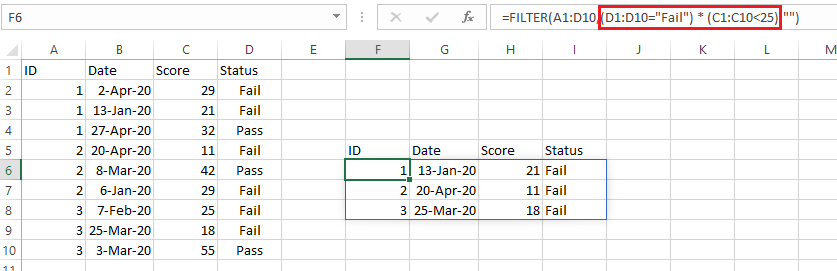## FILTER with OR criteria

Let us consider the example below:The aim is to return an array where the Order Priority is either Critical OR Low

To extract the required result, we can use MATCH function with FILTER:

`=FILTER(A1:C15,ISNUMBER(MATCH(A1:A15,E2:E3,0)),"")`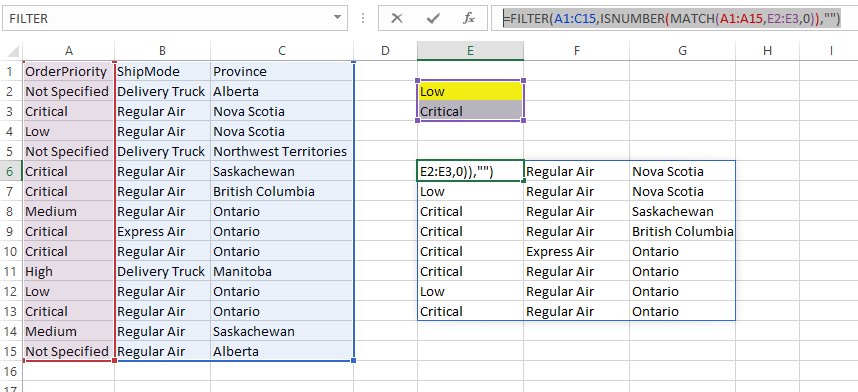Alternatively, we could write the same formula as:

`=FILTER(A1:C15,ISNUMBER(MATCH(A1:A15,{"Critical","Low"},0)),"")`

Using * operator, we can combine multiple OR criteria:

`=FILTER(A1:C15,ISNUMBER(MATCH(A1:A15,E2:E3,0)) * ISNUMBER(MATCH(C1:C15,G2:G3,0)),"")`## Using FILTER with VLOOKUP & XLOOKUP

FILTER function is quite useful when used with VLOOKUP or XLOOKUP. We can return the search result by meeting multiple criteria.

`=VLOOKUP(F4,FILTER(A1:D10,D1:D10 = "Pass",""),3,0)`

` =XLOOKUP(F4,FILTER(A1:A10,D1:D10="Pass",""),FILTER(C1:C10,D1:D10="Pass",""))`This formula returns the result based on ID and Status = "Pass"

@imVivRan